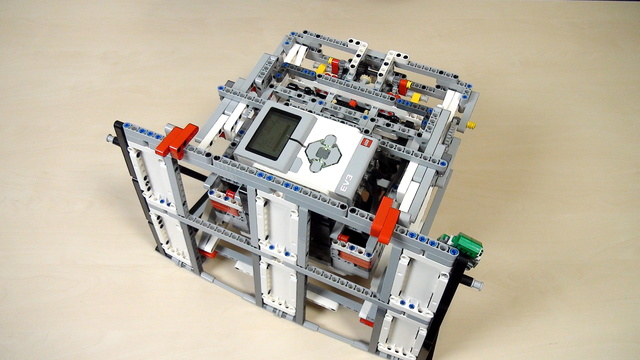# Improving FLL Robot Game. The planetary mechanism for Lifting the robot ProPreview

If you've done the calculation following the previous tutorials you would arrive at a result of 18.75 rotations. But this is not the correct answer. The calculation is wrong, because the math model that we've built, although kind of obvious, is not correct. When experimenting the correct number of rotations would be 37.5. This is a large difference. Two times larger. Exactly two times large. Something should be happening here - and this thing is "planetary mechanism"

• #476
• 12 Apr 2017
• 6:46

### Robot Lifting Attachment With a scissors mechanism

For the FIRST LEGO League 2016-2017 Animal Allies we prepared an attachment for lifting the robot. The idea of the attachment is to show how you could lift the whole robot with a system of gear wheels, levers, and scissors constructions.

Built mainly from LEGO Mindstorms EV3 parts but could probably be constructed from NXT sets.

### English

If you've done the calculation correctly, the correct value is 18.75 for driving this small gear wheel - the driving gear wheel to get to 90 degrees for the large driven gear wheel. The experimental value if you do the experiment correctly, you'll reach a value of 37.5. So, we have 18.75 shown by the mathematical model and 37.5 shown by the experiment. And this is a very large difference. Let's see why. The mathematical model that we built for our system is correct up to the point for taking into account all the ratios between the gears and the gear wheels. But we don't take into account this gear wheel. And this is actually the final driven gear wheel. So, if I take the model and the sheet of paper with the model we have one more wheel that has 40 teeth here and this is actually the final wheel. This is the wheel that drives the scissor mechanism. And there is a relation between these two gear wheels. They are not connected as in a gear system where we can calculate the ratio because the ratio will be 1. But there is another more special relation between the two wheels. So, again the reason for arriving at a different answer and the answer was 37.5. We have 37.5 rotations when doing the experiment and 18.75 mathematically. And you can see that the difference between the two values is actually multiplied by 2. If we multiply 18.75 x 2, we'll get 37.5. And this means that the ratio between this wheel and this wheel is actually 2. But how could that be if both wheels are with 40 teeth? How could there be a relation between them that multiplies the final result by 2?

The simple and very straightforward answer is that here we have a planetary mechanism. A planetary mechanism is a mechanism where one of the gears is rotating around its axle but also around the axle of another wheel. So, in this whole attachment we have the system of gears here that changed the ratio and at the end we have a planetary mechanism that's connected to the scissor mechanism. We have this beam that's actually part of the scissor mechanism and we want to rotate this beam to 90 degrees. But the beam is just connected to the axle and not to the wheels. This means that rotating the wheel to 90 degrees is not equal to rotating the beam to 90 degrees. Actually, we should rotate this wheel to 180 degrees and this will result in the beam rotating to 90 degrees. Now, to enter into details of the planetary mechanism we'll build another resource and we'll try to link it somewhere here in the course below the video so that you can learn more about planetary mechanisms. In this case when you have these two wheels the relation between them is that you should rotate this driving gear wheel to 180 degrees and this will result in the beam rotating to 90 degrees. And why are we skipping this from the course? Because it's a very interesting topic but it's not something that you should check in order to arrive at this attachment. It's is something you can check to understand more about the attachment. But it's not mandatory. It's something like more details that we'll use to describe how the planetary mechanism works.

So, as a result we have 18.75 here as rotations from the driving wheel. This results in 90 degrees rotation of the 40 teeth gear wheel and this will make the beam rotate to just 45 degrees. To make the beam rotate to 90 degrees we should do 37.5 rotations with the driving wheel. Because the relation here is 1 by 2. We multiply by 2. We should divide by 2, then we should multiply the driving wheel by 2. What we have learned here is that we've described some of the losses that occur in the gear system like the gaps between the gears and the staging and other effects that occur but they don't have such a great effect. They have an effect on the whole system but the effect is not that great. So, if something is not working with your calculation, it is probably the calculations that are wrong. And the losses are a small percentage. The losses cannot be 2 times the calculation. And let's try now with 37.5 rotations for the driving wheel.

## Courses and lessons with this Tutorial

This Tutorial is used in the following courses and lessons• 48
• 118:45
• 15• 8
• 0
• 2
• 3d_rotation 1